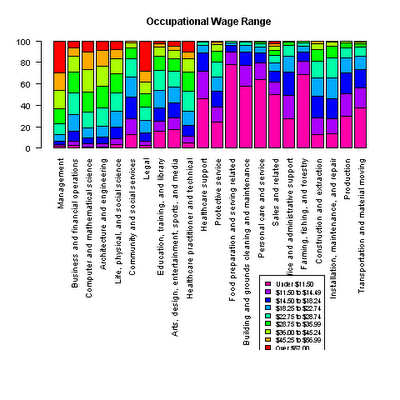Want to share your content on R-bloggers? click here if you have a blog, or here if you don't.

In the previous post I created a chart but could not figure out to fit the legend in the chart area. Peter Carl pointed me to the layout command which partitions the display area and allowed the the legend to be included.Source code to produce the chart above is as follows.

library(XML)
colnames(tb)=c(‘Major occupational group’,
‘Under \$11.50′,’\$11.50 to \$14.49′,’\$14.50 to \$18.24′,’\$18.25 to \$22.74′,’\$22.75 to \$28.74′,’\$28.75 to \$35.99′,’\$36.00 to \$45.24′,’\$45.25 to \$56.99′,’Over \$57.00’)
rownames(tb)=tb[,1]
tb=tb[-1]
tb=sub(‘%’,”,as.matrix(tb))
par(las=2, cex=0.85)

# Here is the new layout command
nf <- layout(matrix(c(1,1,0,2), 2, 2, byrow=TRUE), respect=TRUE)
barplot(t(tb), main=”Occupational Wage Range”, col=rev(rainbow(9)), names.arg=names(tb))

# Created a dummy plot
barplot(t(tb), main=””, col=NA, border=”NA”, axes=FALSE, names.arg=rep(”,length(rownames(tb))))
legend(3, 67.5, colnames(tb), cex=0.8, fill=rev(rainbow(9)))

The layout command divides up the area into a matrix. Calling layout.show() results in the layout being displayed for your reference. This allows you to experiment with the parameter options and immediately get a sense of how a given layout will be rendered.

nf <- layout(matrix(c(1,1,0,2), 2, 2, byrow=TRUE), respect=TRUE)
layout.show(nf)

I did a “dummy plot” in the example above to force the focus to move to the next section of the layout (number 2 above). Is there a better way to make the legend appear in this area?

I plan to port this example to ggplot2 and lattice – from what I can see, base graphics is sort of the least common denominator for plots in R. There are tons of examples available in base graphics, but these other packages generally provide better designed charts that are a bit more aesthetically pleasing. Because these have different APIs, it will take some time to ramp up on the calls required to emulate what I already can do in base graphics. More to learn. More to blog :).